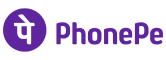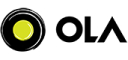New update is available. Click here to update.
Topics

# Program to validate IP address

Easy0/40
Average time to solve is 15m+2 more companies

## Problem statement

Conditions for a valid ‘IPv4’ are:

``````1. Text form of ‘IPAddress’ must be ‘a.b.c.d’
2. The values of a,b,c and d can vary from ‘0’ to ‘255’ and both ‘0’ and ‘255’ are inclusive.
``````
Detailed explanation ( Input/output format, Notes, Images )
Constraints:
``````1 <= T <= 10^4
1 <= length of given text <= 50

Time limit: 1 sec
``````
Sample Input 1:
``````4
123.111.12.k
122.0.330.0
1.1.1.250
1.0.0.0.1
``````
Sample Output 1:
``````False
False
True
False
``````
Explanation of sample input 1:
``````Test Case 1:
Given text ‘IPAddress = 123.111.12.k’, it is satisfying the first condition that given ‘IPAddress’ must be ‘a.b.c.d’ formed but it not satisfying the second condition that d must in a range of ‘0’ to ‘255’ but the value of ‘d’ is ‘k’.
Hence return ‘False’.

Test Case 2:
Given text ‘IPAddress = 122.0.330.0’, it is satisfying the first condition that given ‘IPAddress’ must be ‘a.b.c.d’ formed but it not satisfying the second condition that c must in a range of ‘0’ to ‘255’ but the value of ‘c’ is ‘330’ and it is out of range.
Hence return ‘False’.

Test Case 3:
Given text ‘IPAddress = 1.1.1.250’, it is satisfying the first condition that given ‘IPAddress’ must be ‘a.b.c.d’ formed as well as it satisfying the second condition that a,b,c, and d must in range of ‘0’ to ‘250’.
Hence return ‘True’.

Test Case 4:
Given text ‘IPAddress = 1.0.0.0.1’, it is not satisfying the first condition for valid ‘IPv4’, that text ‘IPAddress’ must be in form of ‘a.b.c.d’ but given text is a form of ‘a.b.c.d.e’
Hence return ‘False’.
``````
Sample Input 2:
``````2
1.90.21.1
1.1
``````
Sample Output 2:
``````True
False
``````Console